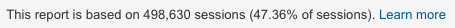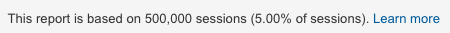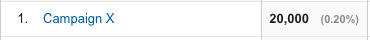# How Accurate Is Sampling In Google Analytics?

### Tags:

March 3, 2016 |You’ve probably seen this little warning in Google Analytics from time to time as you look at reports:When the number is less than 100%, that means that Google Analytics is employing sampling to estimate the total. This occurs when you request a report that isn’t pre-aggregated (by using a segment, adding a secondary dimension, or creating a custom report, for example) and the number of sessions in your data is over 500,000.

(There are some other conditions or reports that will also trigger sampling. You can read more about sampling and some of the ways to overcome it in some of our previous articles on the LunaMetrics blog, and GA Premium is an option to raise the sampling limits if you have large volumes of data.)

But when sampling does occur, what happens? And how accurate is it likely to be? This is actually a relatively straightforward statistical problem that comes up all the time in measuring a sample of a population (in medical studies or political surveys, for example). Let’s take a look.

## Let’s Do the Thing with the Numbers

Here’s an example with nice round numbers to illustrate how sampling works. Suppose we have a site with 10,000,000 sessions for some time period we’re looking at. We’d know this from the Audience Overview report (which is pre-aggregated, so these numbers will always show using 100% of the data—no sampling. (In statistics terms, this is our population size; we’ll call it N.)Now suppose we apply a segment or use a custom report. Since 10,000,000 > 500,000, sampling kicks in.Again, for round numbers, we’ll say that GA is using 500,000 as the sample size. (Typically, you’ll find that this number is a little bit less than 500,000. In statistics terms; this is our sample size, we’ll call it n.) If we take N / n = 10,000,000 / 500,000 = 20, we can find the scaling factor GA uses on the sample to generate totals for the entire population.

For example, suppose there are 1,000 sessions from Campaign X in the sample. GA takes 1,000 x 20 = 20,000 to report the total number of sessions from that channel.But the key realization is that this is just an estimate based on the sample. The real number might be 19,241 or 20,554. The 500,000-session sample is randomly selected from our 10,000,000 sessions. As a result, depending on how the dice fall in selecting the sample, there’s variation. There’s even a VERY TINY chance that our sample included all 1,000 sessions from this campaign in the entire population (so the actual value is 1,000). Or, there’s a VERY TINY CHANCE that the other 9,500,000 sessions in the population are all from this campaign (so the actual value is 9,501,000).

Fortunately, the math can help us establish some likely bounds for the actual value (this is called margin of error). It’s likely that the actual value is somewhere around our estimate of 20,000. We have to make a choice in how sure we want to be—this is called a confidence limit. Typically 95% is used, and that’s what we’ll go with through the rest of our calculations.

The margin of error can tell us a range of likely actual values in the total population (like in survey data when you see “±5%”, for example). And this will give us a better idea of how much weight we give to sampled values we see in the GA interface.

We’re going to look at 3 different scenarios:

• Finding the margin of error in the number of sessions in a particular segment or dimension
• Finding the margin of error in rate-based metrics, such as conversion rate or bounce rate
• Finding the margin of error in measurement metrics, such as session duration, pages per session, or revenue

## Margin of Error for Sessions in a Subset

To calculate our margin of error with 95% confidence limit, we’re going to use the following formula:

p ± 1.96 √(p(1–p)/n)

Where:

• n is the sample size. In our example above, that was 500,000.
• p is the sample proportion, the fraction of the sample that is of interest. In our example above, that was 1,000 / 500,000 = 0.002 (or 0.2%)

(This formula is known as the normal approximation for finding a margin of error, and it works well for large sample sizes. Below we’ll look at an alternative when the sample sizes get smaller.)

For our values, this gives us:

`0.002 ± 0.000124`

If we multiply this by the size of our population (N = 10,000,000), we get the following interval:

`20,000 ± 1,240`

In other words, a range from 18,760 to 21,240. So with the numbers in this particular example, the actual number might be as much as 1,240 sessions higher or lower than the number in GA, with a 95% confidence interval.

Note that there are no guarantees in life when it comes to statistics, no matter how much math we do. With these confidence limits, 95% of the time, a sample will include the actual value in this range, but 5% of the time—or 1 time in 20—it won’t. So for every 20 calculations like this you do, one is wrong, in the long run. You’ve just got to live with it.

### Correction Factor Based on Population Size

You might have noticed that the population size didn’t come into our formula for margin of error—the margin is independent of the size of the population. That’s because the assumption in this formula is that the population is infinite (or at least, much larger than the sample size).

Logically, however, you probably realize that we are more likely to achieve an accurate estimation if we sample 500,000 sessions out of a population of 600,000 vs. a population of 10,000,000. There’s a correction factor we can use on the margin of error to reflect this:

√((N–n)/(N–1)

Here’s a handy table for the correction factor with a sample size of 500,000 with the following population sizes:

```   600,000    0.41    750,000    0.58  1,000,000    0.71  2,000,000    0.87  3,000,000    0.91  4,000,000    0.94  5,000,000    0.95 10,000,000    0.97```

So with our population size of 10,000,000, the correction factor is 0.97. That means rather than 20,000 ± 1,240, our corrected interval would be 20,000 ± 1,207 (not much of a change).

However, if our total sessions in GA was 600,000, the correction factor is 0.41. The uncorrected interval is 20,000 ± 299, but the corrected interval is 20,000 ± 122 (quite a bit narrower than before correction).

## Margin of Error for Rate Metrics

Consider Campaign X we looked at above. If GA says the conversion rate for this campaign is 2%, what’s the margin of error? Rate metrics, like bounce rate or conversion rate, are just a number of sessions for a subset in disguise. They give a sample proportion (p) rather than the number of sessions.

Remember there were only 1,000 sessions from Campaign X. So the sample size, in this case, is 1,000, and the sample proportion is 0.02 (2% conversion rate). This means there were only 0.02 x 1000 = 20 conversions from that campaign in the sample. Now we’re dealing with pretty small numbers. When the numbers get small, the formula we used before (the normal approximation) isn’t as good as some other methods of calculating margin of error. We’ll use a method based on the binomial distribution instead. (If that doesn’t mean anything to you, don’t worry, it’s just more accurate for smaller samples sizes and proportions. And trust me, you don’t even want to see the formula for this one…)

Here our sample size is 1,000 and the sample proportion is 0.02. The value range using the binomial margin of error at the 95% confidence limit is:

`1.23% to 3.07%`

(Note that with the binomial method, the range is not symmetric, so it can’t be expressed as “p ± something”. The normal approximation in this example gives 2% ± 0.87%, or 1.13% to 2.87%. Not a lot different, but with smaller numbers of sessions or conversion rates closer to 0, the differences become more pronounced. The binomial method is a fairly conservative method that guarantees 95% coverage or better; there are other methods you can employ, but they get more complicated to compute.)

So even though Google Analytics says, with this particular sample, Campaign X’s conversion rate is 2%, it could be a lot different. When we’re talking about rates that are small to begin with (as most conversion rates usually are) even a difference of 1% or less can be a lot.

## Margin of Error for Measurement Metrics

If you’re not tired of the math yet, there’s yet another formula for measurement metrics like session duration, pages per session, or revenue. (These metrics are typically expressed as an average of the values for each session, as opposed to the categorical “yes/no” type metrics discussed above: converted or not, bounced or not, in Campaign X or not.)

Unfortunately, this formula is based on two statistics for the metric: the mean (which we have in GA) and the standard deviation (which we do not). To calculate the standard deviation, we’d need each of the individual observed values in the sample. For revenue, we might be able to calculate that information (via the Sales Performance report in the Ecommerce reports), but for other metrics there’s no clean way to do this. So these type of metrics don’t have a straightforward way to calculate a margin of error from Google Analytics.

## I’m Tired of Math and I Just Want to Push the Buttons

OK, fair enough. Here are two calculators that do all that.

### Margin of Error for Sessions in a Category

#### (normal approximation with finite population correction, 95% confidence limits)

total sessions from Audience Overview with no segments applied

from the sampling message at the top of the report of interest

with a segment applied or looking at a row within a report

`The value is ___ ± ___, or ___ to ___ (95% confidence).`

### Margin of Error for Rate Metrics in a Category

#### (binomial method, 95% confidence limits)

total sessions from Audience Overview with no segments applied

from the sampling message at the top of the report of interest

with a segment applied or looking at a row within a report

express as a decimal, e.g. 2% as 0.02

`The value is ___ and within the range of ___ to ___ (95% confidence).`

## Takeaway

The smaller the segment or dimension value is in proportion to the total, the wider the margin of error. Be aware of this and take sampled values with a grain (or shaker) of salt. Use methods to work around sampling if possible.